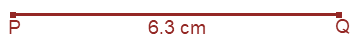# ML Aggarwal Solutions for Class 6 Maths Chapter 13 Practical Geometry

ML Aggarwal Solutions for Class 6 Maths Chapter 13 Practical Geometry, are provided here, so that students can check for the answers, whenever they are facing difficulty while answering the questions. These solutions help students to clear doubts quickly and assist in learning the topic effectively. Students of Class 6 are suggested to practice ML Aggarwal Solutions for Class 6 Maths Chapter 13 to get more marks in the examination.

Chapter 13 – Practical Geometry is based on the study of construction of a line parallel to a given line, through a point not on the line and more topics on Practical Geometry. Students can refer and download ML Aggarwal Solutions PDF from the below mentioned links and can use it for future reference as well.

## Download the PDF of ML Aggarwal Solutions for Class 6 Maths Chapter 13 Practical Geometry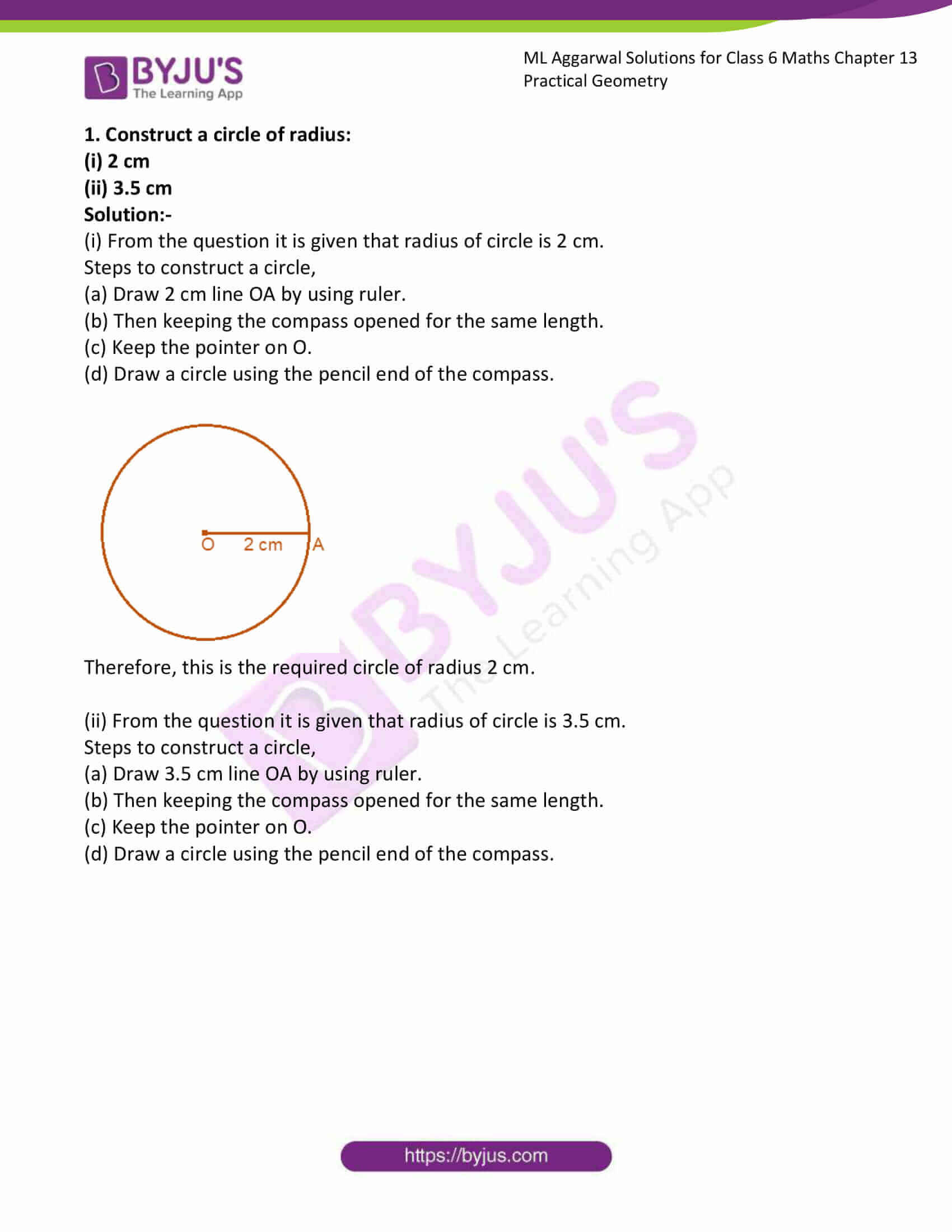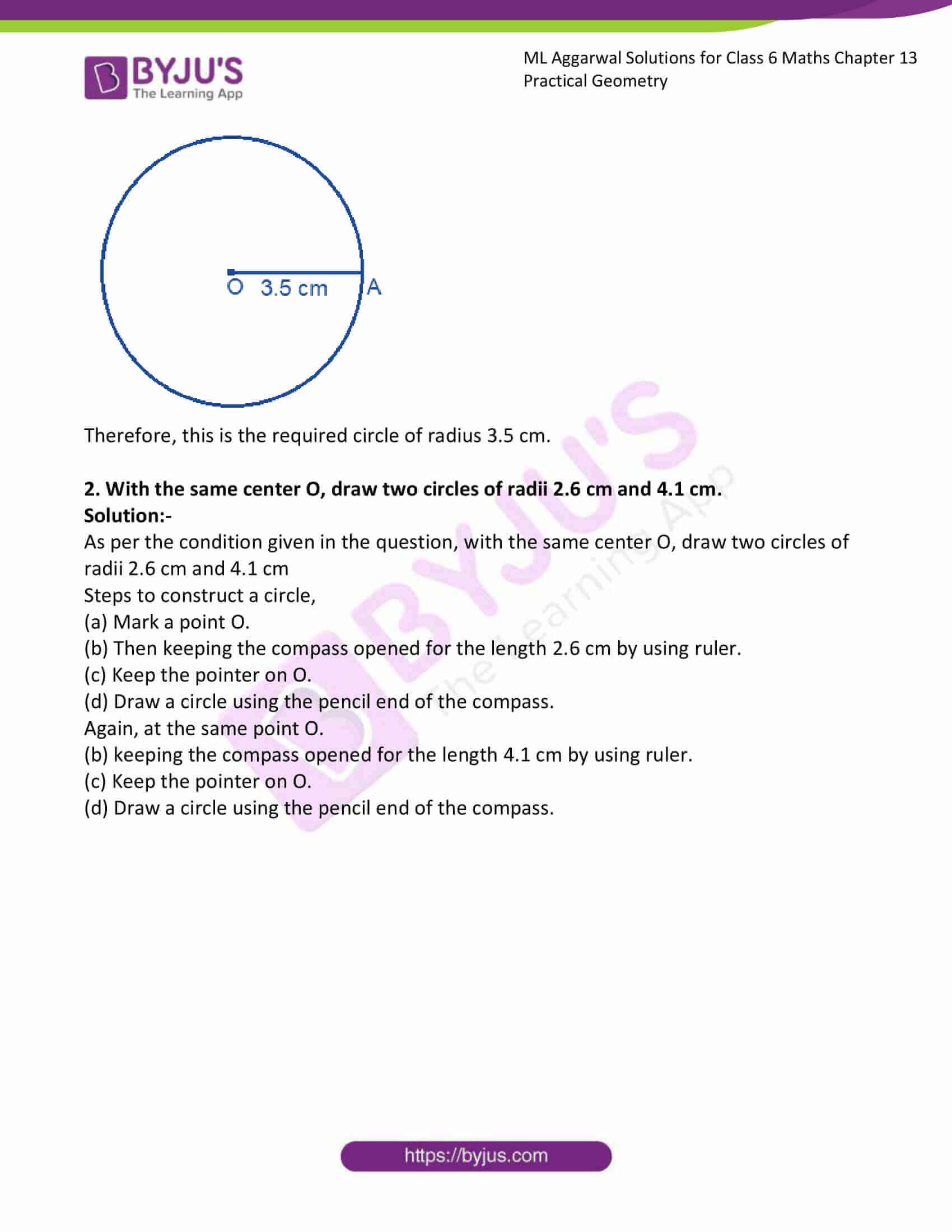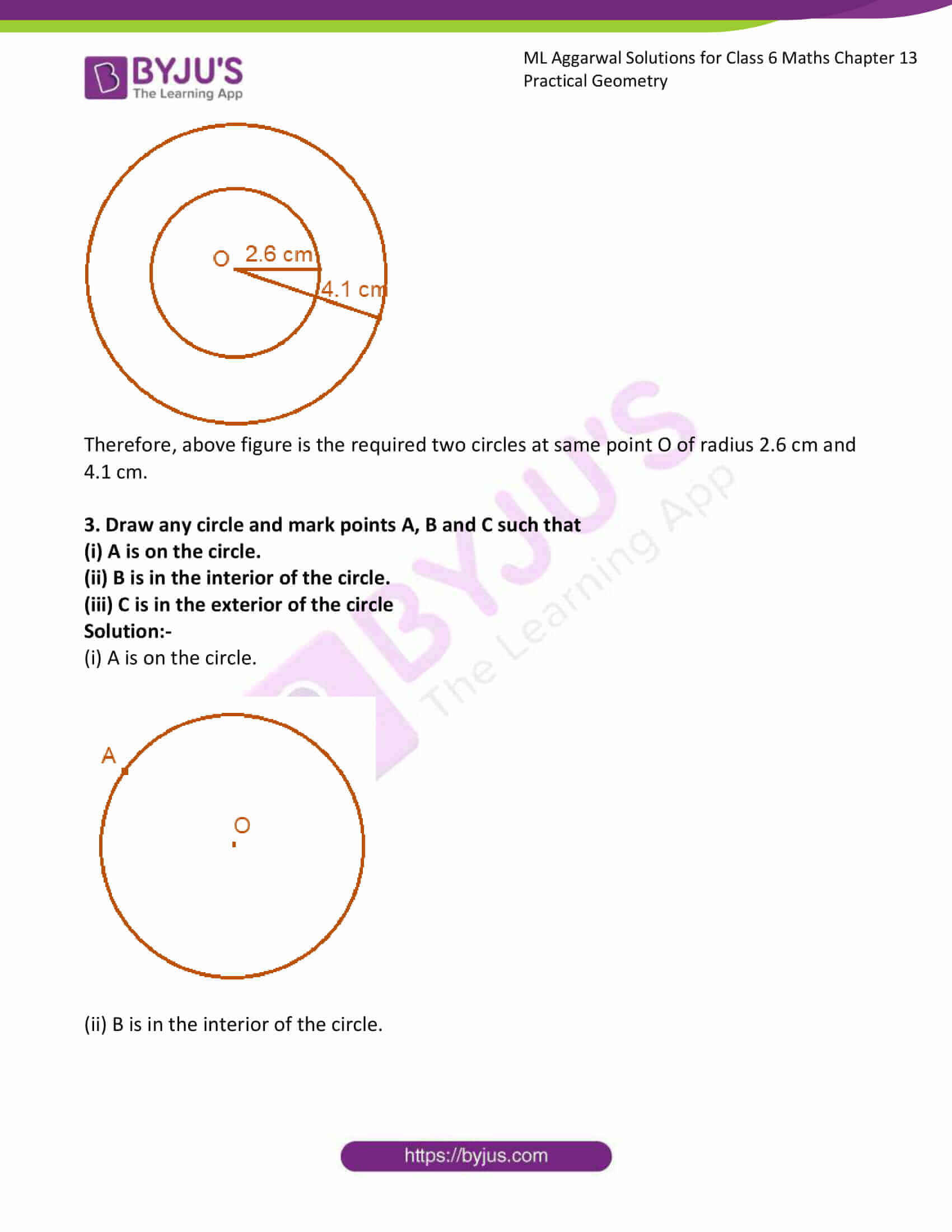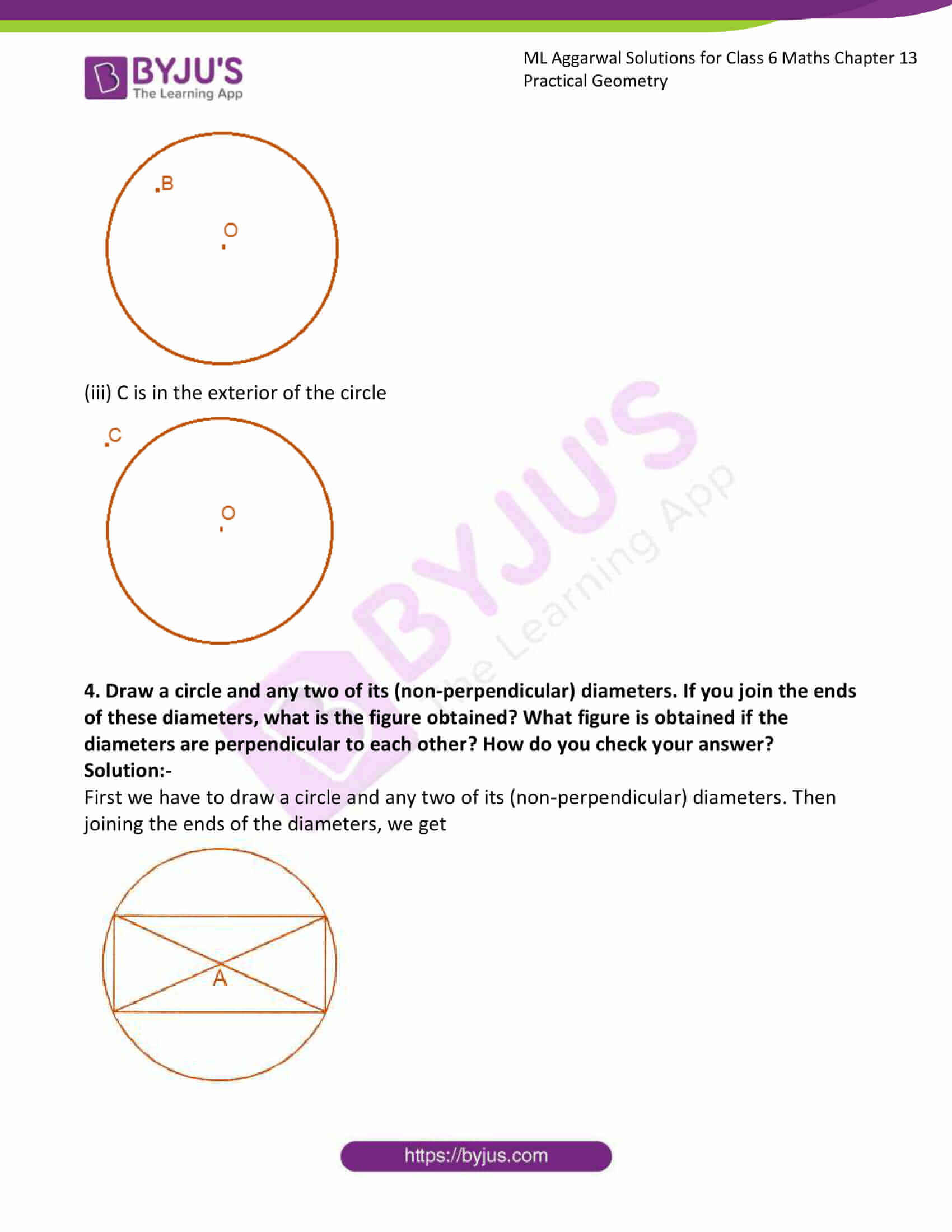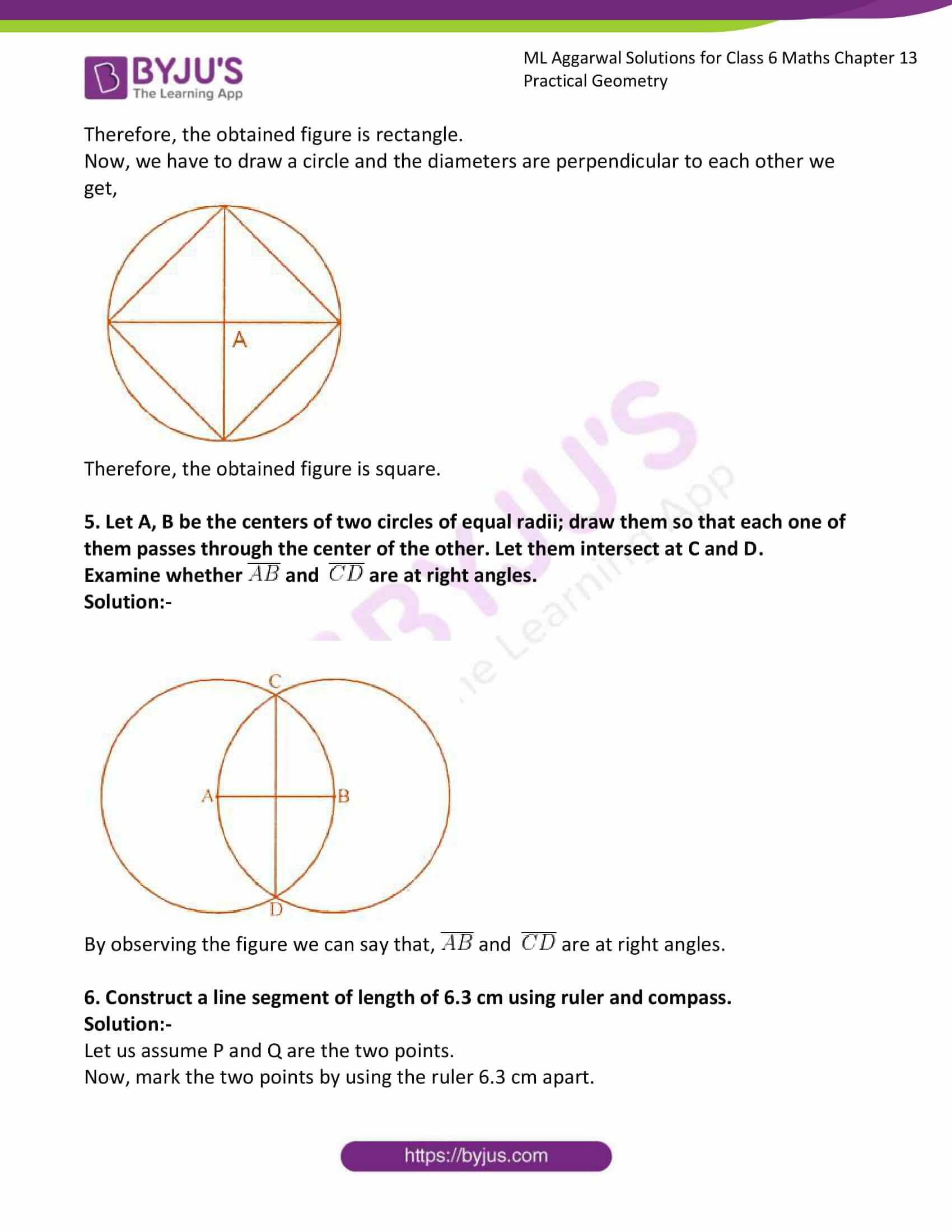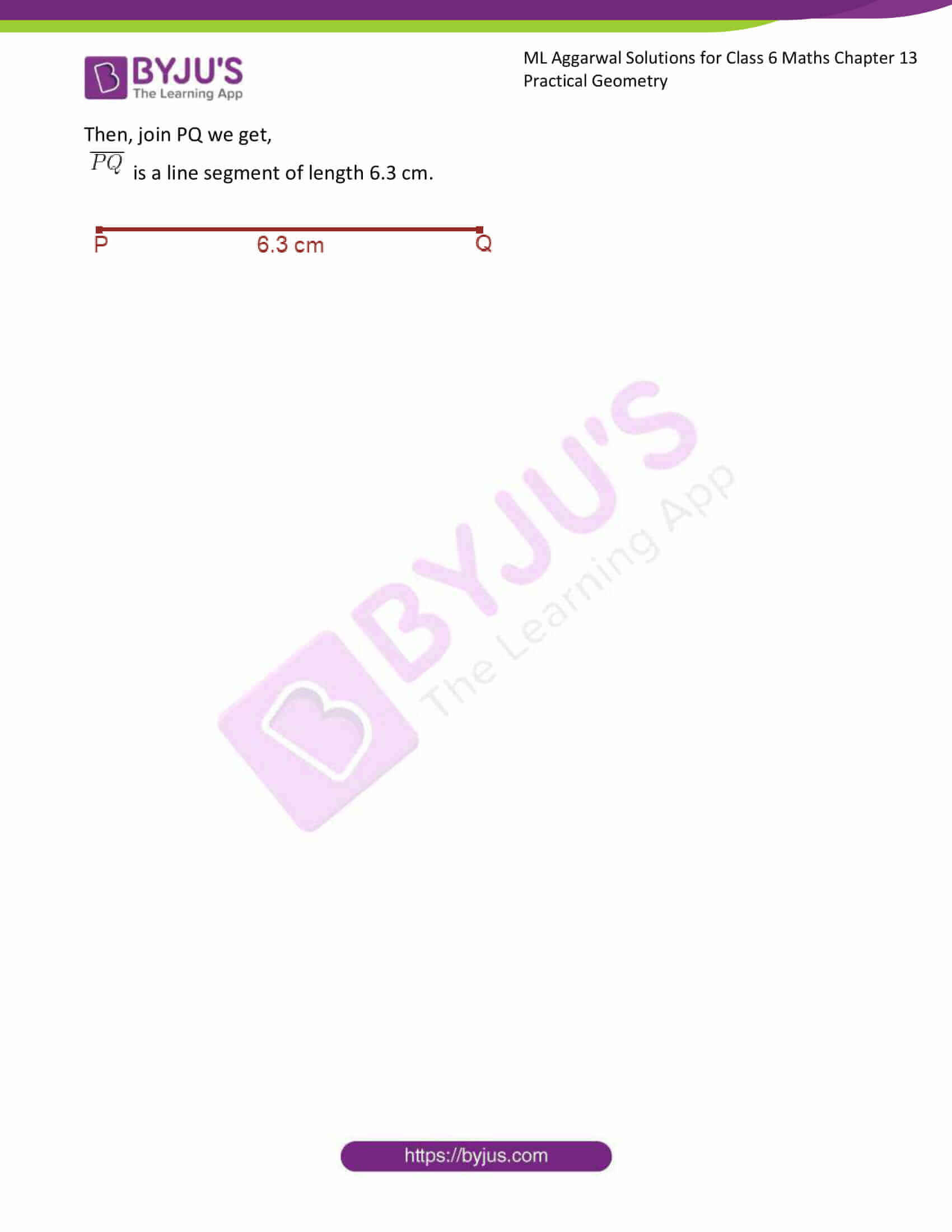### Access answers to ML Aggarwal Solutions for Class 6 Maths Chapter 13 Practical Geometry

1. Construct a circle of radius:
(i) 2 cm
(ii) 3.5 cm

Solution:-

(i) From the question it is given that radius of circle is 2 cm.

Steps to construct a circle,

(a) Draw 2 cm line OA by using ruler.

(b) Then keeping the compass opened for the same length.

(c) Keep the pointer on O.

(d) Draw a circle using the pencil end of the compass.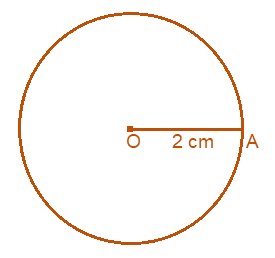Therefore, this is the required circle of radius 2 cm.

(ii) From the question it is given that radius of circle is 3.5 cm.

Steps to construct a circle,

(a) Draw 3.5 cm line OA by using ruler.

(b) Then keeping the compass opened for the same length.

(c) Keep the pointer on O.

(d) Draw a circle using the pencil end of the compass.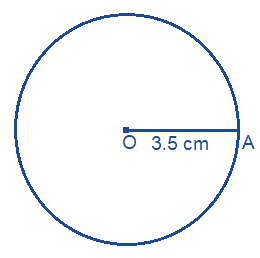Therefore, this is the required circle of radius 3.5 cm.

2. With the same center O, draw two circles of radii 2.6 cm and 4.1 cm.

Solution:-

As per the condition given in the question, with the same center O, draw two circles of radii 2.6 cm and 4.1 cm

Steps to construct a circle,

(a) Mark a point O.

(b) Then keeping the compass opened for the length 2.6 cm by using ruler.

(c) Keep the pointer on O.

(d) Draw a circle using the pencil end of the compass.

Again, at the same point O.

(b) keeping the compass opened for the length 4.1 cm by using ruler.

(c) Keep the pointer on O.

(d) Draw a circle using the pencil end of the compass.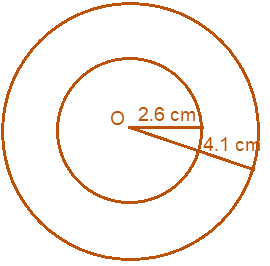Therefore, above figure is the required two circles at same point O of radius 2.6 cm and 4.1 cm.

3. Draw any circle and mark points A, B and C such that
(i) A is on the circle.
(ii) B is in the interior of the circle.
(iii) C is in the exterior of the circle

Solution:-

(i) A is on the circle.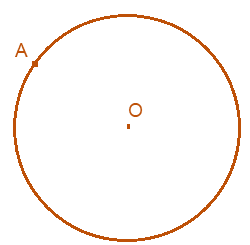(ii) B is in the interior of the circle.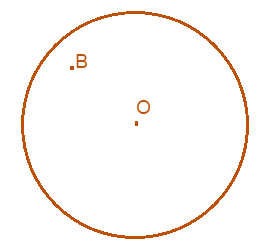(iii) C is in the exterior of the circle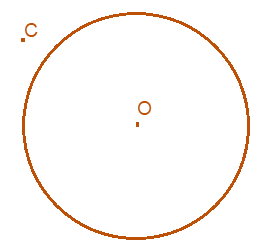4. Draw a circle and any two of its (non-perpendicular) diameters. If you join the ends of these diameters, what is the figure obtained? What figure is obtained if the diameters are perpendicular to each other? How do you check your answer?

Solution:-

First we have to draw a circle and any two of its (non-perpendicular) diameters. Then joining the ends of the diameters, we get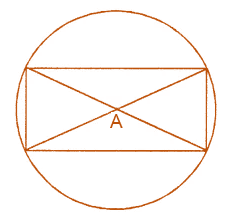Therefore, the obtained figure is rectangle.

Now, we have to draw a circle and the diameters are perpendicular to each other we get,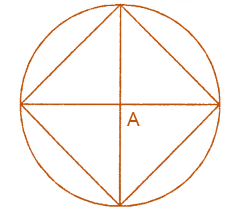Therefore, the obtained figure is square.

5. Let A, B be the centers of two circles of equal radii; draw them so that each one of them passes through the center of the other. Let them intersect at C and D.

Examine whether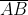and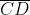are at right angles.

Solution:-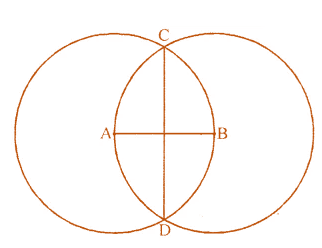By observing the figure we can say that,andare at right angles.

6. Construct a line segment of length of 6.3 cm using ruler and compass.
Solution:-

Let us assume P and Q are the two points.

Now, mark the two points by using the ruler 6.3 cm apart.

Then, join PQ we get,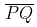is a line segment of length 6.3 cm.